Courses

# Test: Gaseous State - 4

## 20 Questions MCQ Test Physical Chemistry | Test: Gaseous State - 4

Description
This mock test of Test: Gaseous State - 4 for Chemistry helps you for every Chemistry entrance exam. This contains 20 Multiple Choice Questions for Chemistry Test: Gaseous State - 4 (mcq) to study with solutions a complete question bank. The solved questions answers in this Test: Gaseous State - 4 quiz give you a good mix of easy questions and tough questions. Chemistry students definitely take this Test: Gaseous State - 4 exercise for a better result in the exam. You can find other Test: Gaseous State - 4 extra questions, long questions & short questions for Chemistry on EduRev as well by searching above.
QUESTION: 1

### Maximum number of electrons in a subshell with l = 3 and n = 4 is

Solution:

n = 4, l  =3, which denotes 4f subshell.
In f subshell, there are 7 orbitals and each orbital can accommodate a maximum of two electrons, so, maximum no. of electrons in 4f subshell = 7 × 2 = 14

Formula to find out the no. of electrons = 4l+ 2
∵ l= 3
⇒ 4 × 3 + 2 = 14

Thus, there are 14 electrons.

QUESTION: 2

### Among the following curves, which is not according to Charle’s law:

Solution:

According to Charles Law, V ∝ T.
i.e. V=kT, where k is proportionality constant.

For A: Charles law is in the format of y=mx
Therefore it is a correct representation.
For B: on taking log on both sides in Charles law we get, log V= log T + log k
i.e. y = mx + c, where m = 1 = tan 45º
Therefore it is a correct representation.
For C: on multiplying with T on both sides we get, VT ∝ T2
Therefore it is a correct representation.
For D: ∵ V/T = constant
Therefore it is an incorrect representation.

QUESTION: 3

### The electronic configuration of a dipositive metal M2+ is 2, 8, 14 and its atomic weight is 56 a.m.u. The number of neutrons in its nuclei would be

Solution: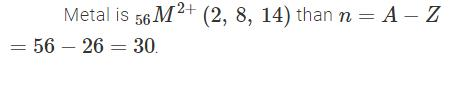QUESTION: 4

Which in not correct curve for gay-lussac’s law:

Solution:

According to Gay-Lussac's Law, P ∝ T.
i.e. P =kT, where k is proportionality constant.

For A: On taking log on both sides in Gay-Lussac's Law we get, log P= log T + log k
i.e. y = mx + c, where m = 1 = tan 45º
Therefore it is a correct representation.
For B: ∵ P/T = constant
Therefore it is an incorrect representation.
For C: ∵ P/T = constant
Therefore it is a correct representation.
For D: Gay-Lussac's Law is in the format of y=mx
Therefore it is a correct representation.

QUESTION: 5

Which is incorrect curve for Boyle’s law:

Solution:

According to Boyle's Law, P ∝ 1/V.

For A: Boyle's law is in the format of y=m/x
Therefore it is a correct representation.
For B: On taking log on both sides in Boyle's law we get, log P= - log V + log k
i.e. y = mx + c, where m = -1 = tan 135º
Therefore it is a correct representation.
For C: Boyle's law is in the format of y=m/x
Therefore it is an incorrect representation.
For D: ∵ V/T = constant
Therefore it is a correct representation.

QUESTION: 6

The energy of an electron in first Bohr orbit of H-atom is –13.6 eV. The possible energy value of electron in the excited state of Li2+ is

Solution: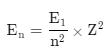where

E1​=Energy of first Bohr orbit =−13.6eV

n= no of orbit

Z=Atomic no

For Li2+, the excited state, n=2 and Z=3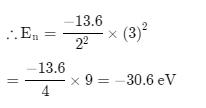QUESTION: 7

Which of the following curve does not represent gay lusacc’s law:

Solution:

P = KT
P = K(tC+273)

QUESTION: 8

For the gaseous reaction, the rate is often expressed in terms of dp/dt instead of dC/dt or dn/dt. What is the relationship among these three expression:

Solution:

∵ C = n/V
on differentiating both sides,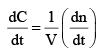According to Ideal gas Equation, PV = nRT
Substituting value of n gives,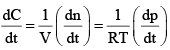QUESTION: 9

A gas can be liquefied most suitably at:

Solution:

For liquefaction of gas temperature should be lower than critical temperature. Also higher is the P in comparison to critical pressure easier is liquefaction .

QUESTION: 10

For a closed (not rigid) container containing n = 10 moles of an ideal gas fitted with a movable, frictionless, weightless piston operating such that pressure of gas remains constant at 0.821 atm, which graph represents the correct variation of log V vs log T where V is in litre and T in Kelvin:

Solution:

According to Charles Law, V ∝ T.
i.e. V=kT, where k is proportionality constant.
On taking log on both sides in Charles law we get, log V= log T + log k
i.e. y = mx + c, where m = 1 = tan 45º.
On comparing with the Ideal gas equation,
k = V/T = nR/P
substituting values,
k = (10 * 0.0821)/0.821 = 1

⇒ log V= log T + log 1
i.e. log V= log T

QUESTION: 11

At a definite temperature (T), the distribution of velocities is given by the curve. The curve that indicates that the velocity corresponding to points A, B and C are: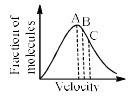Solution:

Vrms = √3kT/m
Vavg = √8kT/πm
Vmp = √2kT/m
√3 > √8\π > √2 (Vrms > Vavg > Vmp)

Speed increases from left to right on the x-axis. Therefore, the root means square speed is farthest to the right on the graph (means it is largest) and the most probable speed is farthest to the left (means that is small).

QUESTION: 12

A graph is plotted between P (atm) vs t°C for 10 mol of an ideal gas as follows: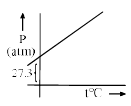Then slope of curve and volume of container (L) respectively, is

Solution:

y−axis =P (atm), x−axis =T (ºC)
Equation of the line,
​P = mT + C
at T = 0, P = 27.3 atm
⇒ C = 27.3
∴ P = mT + 27.3 ...(i)

Ideal gas equation (T in K): PV = nRT
Volume at 0 ºC,
27.3 × V = 10 × 0.0821 × 273
⇒ V = 8.21 Litres

Ideal gas equation in (T in ºC):
PV = nR (T+273)
n = 10, R = 0.0821, V = 8.21
P = (nR/V) T + (nR/T) × 273
Substituting values,
P = 0.1 T + 27.3 ...(ii)
Comparing (ii) with (I)
Slope = m = 0.1

QUESTION: 13

For two samples A and B of ideal gas following curve is plotted between n vs V (volume) of container) at 16.42 atm pressure as follows, then temperature of A and B respectively are: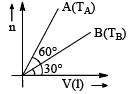Solution:
QUESTION: 14

Match the Column-I and Column-II: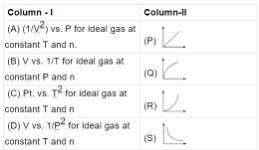Solution:

Correct Answer :- D

Explanation : A-Q, B-S, C-P, D-R

QUESTION: 15

Match the correct from List-1 to List-2 on the basic of following Andrews isotherm of Real gas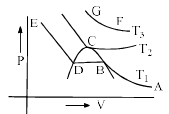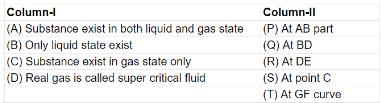Solution:

Refer to the following diagram and compare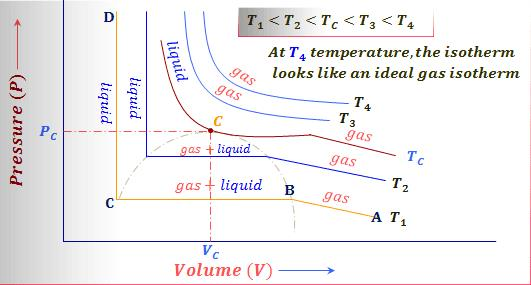QUESTION: 16

Match the Colum –I and Column-II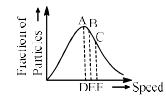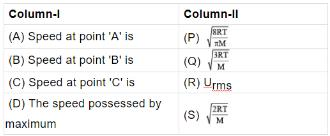Fraction of the gas particles

Solution:
QUESTION: 17

Initial temperature of an ideal gas is 75°C. At what temperature, the sample of neon gas would be heated to double its pressure, it the initial volume of gas is reduced by 15%:

Solution:
QUESTION: 18

At constant volume for a fixed number of moles of a gas, the pressure of the gas increases with the rise in temperature due to:

Solution:
• Pressure on the walls of the container is equal to the change of momentum per unit time per unit area.
• At constant volume, for a fixed number of moles of a gas, the pressure increases with rise in temperature due to an increase in average molecular speed.
• This increases the change in momentum during collisions.
QUESTION: 19

Three flasks of equal volumes contain CH4, CO2, and Cl2 gases respectively. They will contain equal number of molecules if:

Solution:

Avogadro's Law
At same condition of temperature and pressure, equal volumes of different gases contain an equal number of molecules.

QUESTION: 20

At 0°C and one atm pressure, a gas occupied 100 cc. If the pressure is increased to one and a halftime and temperature is increased by one-third of absolute temperature, then final volume of the gas will be:

Solution:

Initial pressure P1 = 1atm
Initial volume V1​ = 100cc
Final temperature T2 ​= (1 + 1 / 3) × 273.15 = 364.2K
Final pressure P2 =  1½ ​× 1 = 1.5atm
Final volume V2 ​= ??
P1​V1 / T1 ​​= T2​P2​ / V2​​
1atm × 100cc / 273.15K ​= 1.5atm × V2 / 364.2K​​
V2 ​= 88.9cc
Hence, the final volume of the gas will be 88.9 cc.